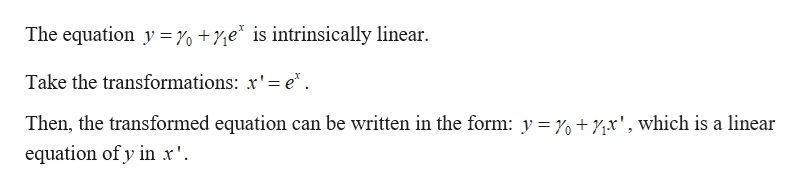Maggenifh 2021-11-19 Answered

The equation $$\displaystyle{y}={y}_{0}+{y}_{1}{e}{x}$$ is an intrinsically linear equation..

• Questions are typically answered in as fast as 30 minutes

Solve your problem for the price of one coffee

• Math expert for every subject
• Pay only if we can solve itromancemrf

Power transformation:
A power transformation or a Box-Cox transformation is a transformation done on the predictor and/or the response variable, by raising the variable to any positive or negative power, which maybe any non-zero integer or fraction, or by taking a logarithm of the variable.Hence, the statement is True.